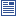Accessibility options:

# First order differential equations resources

Show me all resources applicable to

### Community Project (1)First Order Ordinary Differential Equations (SOURCE)
A zip file containing the LaTex source files and metatdata for the Teach Yourself resource First Order Differential Equations: A summary of five common methods to solve first order ODEs: direct integration, separation of variables, homogeneous equations, integrating factor and Bernouilli equations. This resource is contributed to the mathcentre Community Project by Morgiane Richard, University of Aberdeen and is reviewed by Shazia Ahmed, University of Glasgow.

### Teach Yourself (1)First Order Ordinary Differential Equations
First Order Differential Equations: A summary of five common methods to solve first order ODEs: direct integration, separation of variables, homogeneous equations, integrating factor and Bernouilli equations. This Teach Yourself resource is contributed to the mathcentre Community Project by Morgiane Richard, University of Aberdeen and is reviewed by Shazia Ahmed, University of Glasgow.

### Test Yourself (2)First order differential equations - Numbas
9 questions on first order differential equations. Straight forward integration (2), separating variables (4), linear (1), homogenous (2). All are either initial value or boundary value problems. Numbas resources have been made available under a Creative Commons licence by the School of Mathematics & Statistics at Newcastle University.First order differential equations - Numbas
9 questions on first order differential equations. Straight forward integration (2), separating variables (4), linear (1), homogenous (2). All are either initial value or boundary value problems. Numbas resources have been made available under a Creative Commons licence by Bill Foster and Christian Perfect, School of Mathematics & Statistics at Newcastle University.